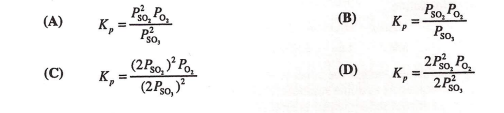# Problem: Consider this reaction.2 SO3 (g) ⇌ 2 SO2 (g) + O2 (g)What is the correct Kp expression for this reaction?

###### FREE Expert Solution
94% (151 ratings)
###### Problem Details

Consider this reaction.

2 SO(g) ⇌ 2 SO(g) + O(g)

What is the correct Kp expression for this reaction?What scientific concept do you need to know in order to solve this problem?

Our tutors have indicated that to solve this problem you will need to apply the Equilibrium Expressions concept. If you need more Equilibrium Expressions practice, you can also practice Equilibrium Expressions practice problems.

What is the difficulty of this problem?

Our tutors rated the difficulty ofConsider this reaction.2 SO3 (g) ⇌ 2 SO2 (g) + O2 (g)What is...as medium difficulty.

How long does this problem take to solve?

Our expert Chemistry tutor, Sabrina took 2 minutes and 30 seconds to solve this problem. You can follow their steps in the video explanation above.

What professor is this problem relevant for?

Based on our data, we think this problem is relevant for Professor Nobile's class at Marquette University.